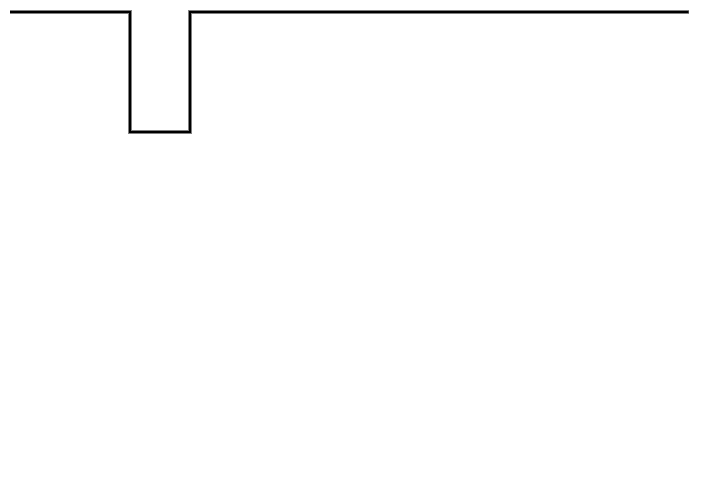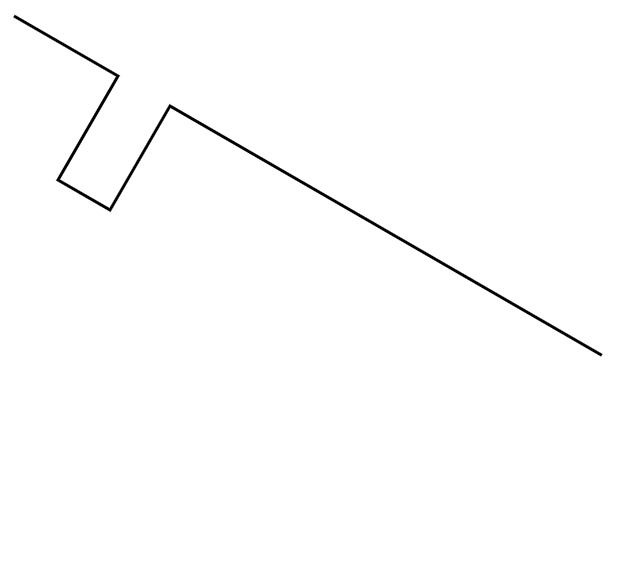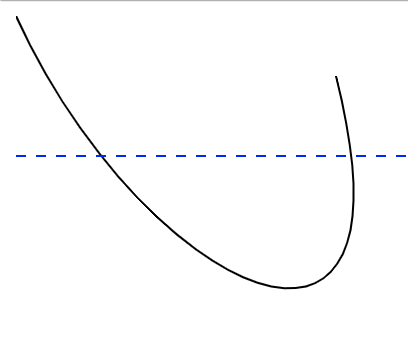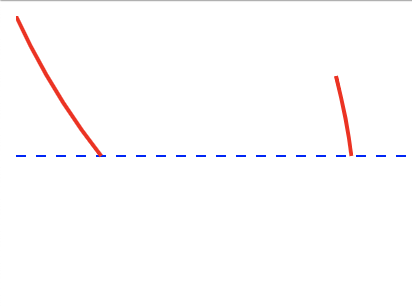# Path Transforms¶

## join¶

the join transform will join all segments into a continuous path. It will make a best effort to rearrange segments to make the smoothest path. For breaks in paths, the segments are extended until they meet.

Attributes

• `close_path`:
• `true` should the final segment be extended to

the meet the beginning of the path?

• `false`

```"transforms" : [
{
"type" : "join",
"close_path" : false
}
]
```

## matrix¶

A matrix transform. Performs an affine transform on all the points within the path.

• `a`

• `b`

• `c`

• `d`

• `e`

• `f`

```"transforms" : [
{
"type" : "matrix",
"a" : 1,
"b" : 0,
"c" : 0,
"d" : 1,
"e" : 1,
"f" : 1
}
]
```

## mirror¶

Mirror will flip the path on either the horizontal or vertical axis

• `axis`:
• `horizontal` will rotate around a horizontal line. Note that this means

the bottom of the shape will become the top.

• `vertical` rotate around a vertical line. This means the

left of the shape will become the right

• `handle`: Defines where on the shape the axis should pass through

```"transforms" : [
{
"type" : "mirror",
"axis" : "horizontal",
"handle" : "\$TOP_LEFT"
}
]
```

## rotate¶

rotate will rotate by the specified amount

• `degrees`: amount to rotate in degrees

• `axis`: The axis point. This will be one of the HANDLE constants. Default is `\$TOP_LEFT`

```"transforms" : [
{
"type" : "rotate",
"degrees" : 30
}
]
```

BEFORE:AFTER:## scale¶

Scale will shrink or expand the path

• `width`: Will scale to the requested width. if `height` is not provided then the scaling

will be proportional

• `height`: Will scale to the requested height. if `width` is not provided then the scaling

will be proportional

• `scale_x`: Percentage to scale in the x direction, where 1 is no change, and .5 would be 50%

• `scale_y`: Percentage to scale in the y direction, where 1 is no change, and .5 would be 50%

```"transforms" : [
{
"type" : "scale",
"width" : 2.1
}
]
```

## slice¶

Slice will slice the component horizontally at the requested Y value and discard everything below that.

• `y`: The Y value to remove all segments below this line.

(Note below here means with a Y value > y, but appears below)

```"transforms" : [
{
"type" : "slice",
"y" : 119
}
]
```

BEFORE:AFTER:## trim¶

trim will remove any surrounding whitespace and move the bounding box of the path to 0,0

## cleanup¶

Some basic path cleanup, the rendered path should not change in any way. This will remove redundant Move operations and some other tasks